## Saturday, 9 August 2014

### CHAPTER 8 - Miscellaneous Techniques

Not all questions can be subjected to the method(s) described earlier. For example, consider the sum$S$ given by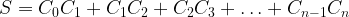$S = {C_0}{C_1} + {C_1}{C_2} + {C_2}{C_3} +\ldots + {C_{n - 1}}{C_n}$
Let us first go through a combinatorial approach, using the observation that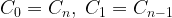${C_0} = {C_n},\;{C_1} = {C_{n - 1}}$ and so on, so that$S$ can be rewritten as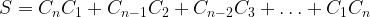$S = {C_n}{C_1} + {C_{n - 1}}{C_2} + {C_{n - 2}}{C_3} +\ldots + {C_1}{C_n}$
Consider a general term of this sum, which is of the form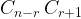${C_{n - r}}\,{C_{r + 1}}$. We can think of this as the number of ways of selecting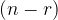$(n - r)$ boys from a group of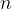$n$boys and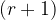$(r + 1)$ girls from a group of$n$ girls. The total number of people we are thus selecting is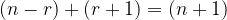$(n - r) + (r + 1) = (n + 1)$. Therefore,$S$ represents the total number of ways of selecting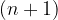$(n + 1)$ people out of a group of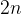$2n$, so that$S$ is simply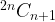$^{2n}{C_{n + 1}}$.
Now to a binomial approach. This will involve generating the general term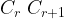${C_r}\;{C_{r + 1}}$ somehow, which is the same as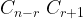${C_{n - r}}\;{C_{r + 1}}$. Consider the general expansion of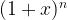${(1 + x)^n}$.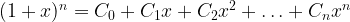${(1 + x)^n} = {C_0} + {C_1}x + {C_2}{x^2} + \ldots + {C_n}{x^n}$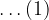$\ldots(1)$
We have to have the terms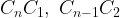${C_n}{C_1},\;{C_{n - 1}}{C_2}$ and so on, which suggests that we write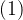$(1)$ twice, but in the second expansion we reverse the terms, multiply, and see what terms contain the (combinations of) coefficients we require.Multiplying, we find on the left hand side we have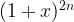${(1 + x)^{2n}}$, while on the right hand side, the terms containing the (combinations of) coefficients we want will always be of the form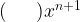$(\,\,\,\,\,\,\,\,\,){x^{n + 1}}$, that is, the power of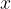$x$ will be$(n + 1)$. No other terms will contain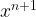${x^{n + 1}}$, verify this for yourself. Thus, the sum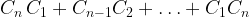${C_n}\,{C_1} + {C_{n - 1}}{C_2} + \ldots + {C_1}{C_n}$ is actually the total coefficient of${x^{n + 1}}$ on the right hand side, and from the left hand side we know that the coefficient of${x^{n + 1}}$ would be simply$^{2n}{C_{n + 1}}$. Thus,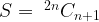$S = {\;^{2n}}{C_{n + 1}}$
A very similar approach could have beenThus,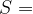$S=$ Coefficient of$x$ in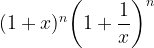${(1 + x)^n}{\left( {1 + \dfrac{1}{x}} \right)^n}$$=$ Coefficient of$x$ in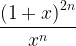$\dfrac{{{{(1 + x)}^{2n}}}}{{{x^n}}}$$=$ Coefficient of${x^{n + 1}}$ in${(1 + x)^{2n}} = {\;^{2n}}{C_{n + 1}}$
 Example: 9
Find the sum$S$ given by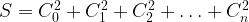$S = C_0^2 + C_1^2 + C_2^2 + \ldots + C_n^2$
 Solution: 9
Note that$S$ can be rewritten as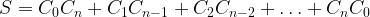$S = {C_0}{C_n} + {C_1}{C_{n - 1}} + {C_2}{C_{n - 2}} + \ldots + {C_n}{C_0}$
Using a combinatorial approach, the sum should be immediately obvious to the alert reader as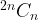$^{2n}{C_n}$. In brief, this is because the right hand side represents, as an example, the total number of ways of selecting$n$ people from a group of$n$ boys and$n$ girls, etc.
Now, we discuss the binomial expansion approach:Thus, we observe that the required sum is the coefficient of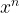${x^n}$ in${(1 + x)^{2n}}$, which is simply$^{2n}{C_n}$.
 Example: 10
Find the sum$S$ given by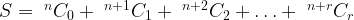$S = {\;^n}{C_0} + {\;^{n + 1}}{C_1} + {\;^{n + 2}}{C_2} +\ldots + {\;^{n + r}}{C_r}$
 Solution: 10
We have already evaluated this sum in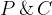$P\, \&\, C$; here we’ll use a binomial approach. Note that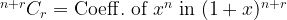$^{n + r}{C_r} = {\rm{Coeff}}{\rm{.}}\;{\rm{of}}\;{x^n}\;{\rm{in}}\;{(1 + x)^{n + r}}$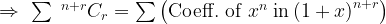$\Rightarrow\,\, \sum {\;^{n + r}}{C_r} = \sum \left( {{\rm{Coeff}}{\rm{.}}\;{\rm{of}}\;{x^n}\;{\rm{in}}\,{{(1 + x)}^{n + r}}} \right)$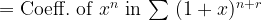${\rm{ = }}\;{\rm{Coeff}}{\rm{.}}\;{\rm{of}}\;{x^n}\;{\rm{in}}\,\sum \,{(1 + x)^{n + r}}$
Thus,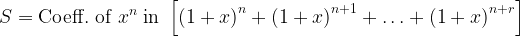$S = {\rm{Coeff}}{\rm{.}}\;{\rm{of}}\;{x^n}\;{\rm{in}}\;\left[ {{{(1 + x)}^n} + {{(1 + x)}^{n + 1}} + \ldots + {{(1 + x)}^{n + r}}} \right]$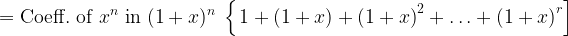$= {\rm{Coeff}}{\rm{.}}\;{\rm{of}}\;{x^n}\;{\rm{in}}\;{(1 + x)^n}\;\left\{ {\left. {1 + (1 + x) + {{(1 + x)}^2} + \ldots + {{(1 + x)}^r}} \right]} \right.$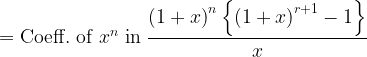$= {\rm{Coeff}}{\rm{.}}\;{\rm{of}}\;{x^n}\;{\rm{in}}\;\dfrac{{{{(1 + x)}^n}\left\{ {{{(1 + x)}^{r + 1}} - 1} \right\}}}{x}$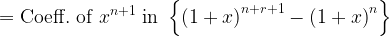$= {\rm{Coeff}}{\rm{.}}\;{\rm{of}}\;{x^{n + 1}}\;{\rm{in}}\;\left\{ {{{(1 + x)}^{n + r + 1}} - {{(1 + x)}^n}} \right\}$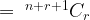$= {\;^{n + r + 1}}{C_r}$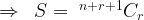$\Rightarrow \,\,\,S = {\;^{n + r + 1}}{C_r}$ which is the same result we obtained in$P\, \&\, C$